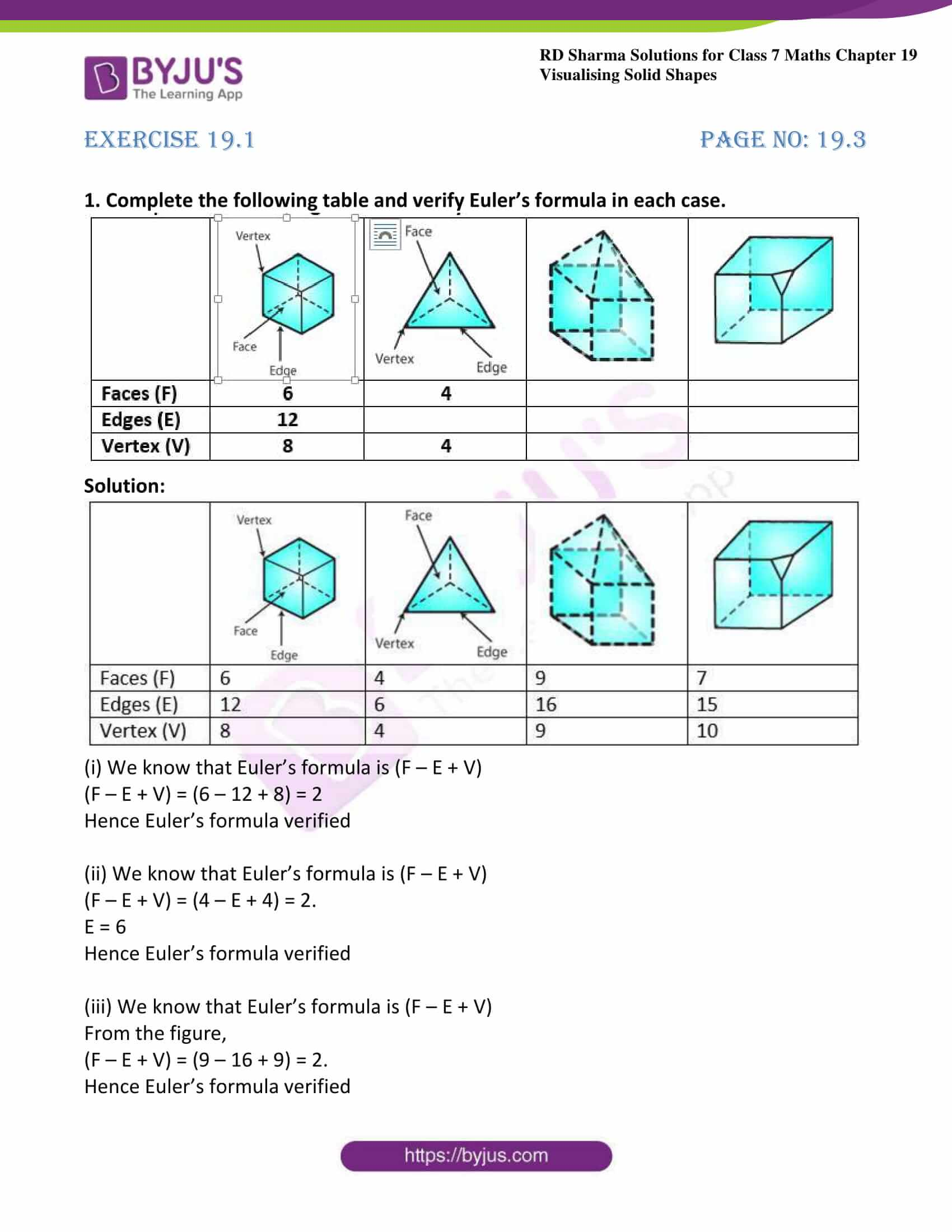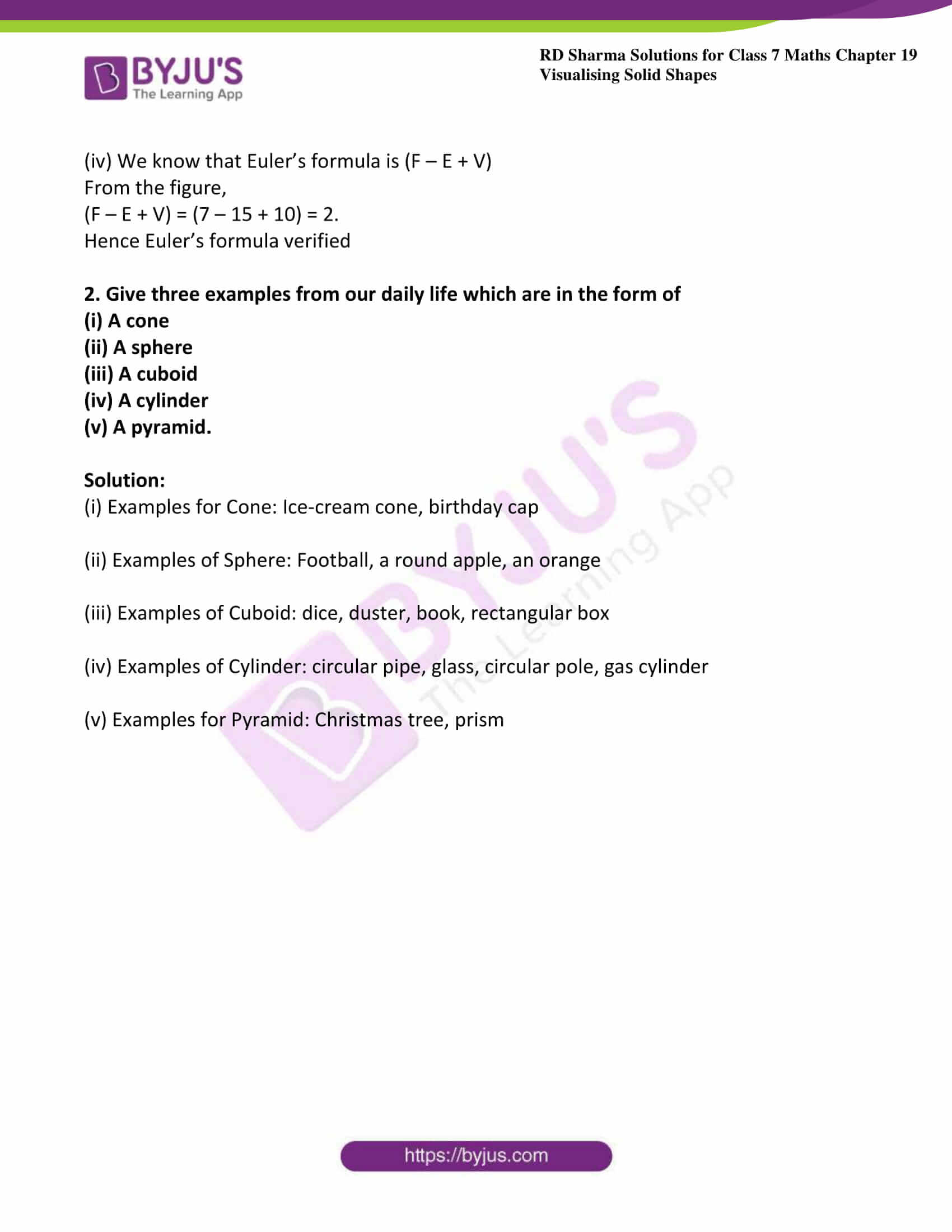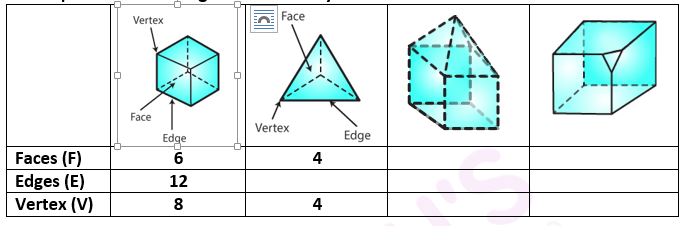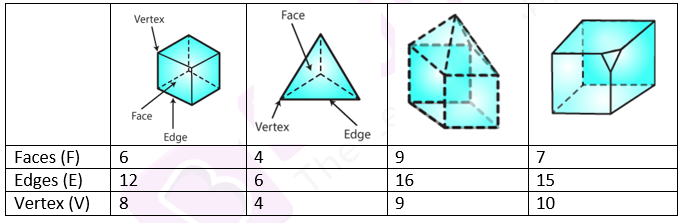# RD Sharma Solutions For Class 7 Maths Exercise 19.1 Chapter 19 Visualising Solid Shapes

In this exercise, we shall study about faces, edges and vertices of three-dimensional figures and applications of Euler’s formula. The RD Sharma Solutions for Class 7 is prepared by our expert tutors at BYJU’S, which is one of the best reference materials to prepare for exams and also helps students to write perfect answer over the subject to score well in their exams. Students can download the pdf from the links given below.

## Download the PDF of RD Sharma Solutions For Class 7 Maths Chapter 19 – Visualising Solid Shapes Exercise 19.1### Access answers to Maths RD Sharma Solutions For Class 7 Chapter 19 – Visualising Solid Shapes Exercise 19.1

1. Complete the following table and verify Euler’s formula in each case.Solution:(i) We know that Euler’s formula is (F – E + V)

(F – E + V) = (6 – 12 + 8) = 2

Hence Euler’s formula verified

(ii) We know that Euler’s formula is (F – E + V)

(F – E + V) = (4 – E + 4) = 2.

E = 6

Hence Euler’s formula verified

(iii) We know that Euler’s formula is (F – E + V)

From the figure,

(F – E + V) = (9 – 16 + 9) = 2.

Hence Euler’s formula verified

(iv) We know that Euler’s formula is (F – E + V)

From the figure,

(F – E + V) = (7 – 15 + 10) = 2.

Hence Euler’s formula verified

2. Give three examples from our daily life which are in the form of

(i) A cone

(ii) A sphere

(iii) A cuboid

(iv) A cylinder

(v) A pyramid.

Solution:

(i) Examples for Cone: Ice-cream cone, birthday cap

(ii) Examples of Sphere: Football, a round apple, an orange

(iii) Examples of Cuboid: dice, duster, book, rectangular box

(iv) Examples of Cylinder: circular pipe, glass, circular pole, gas cylinder

(v) Examples for Pyramid: Christmas tree, prism